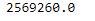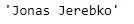Related Articles

# Python | Pandas dataframe.get_value()

• Last Updated : 19 Nov, 2018

Python is a great language for doing data analysis, primarily because of the fantastic ecosystem of data-centric python packages. Pandas is one of those packages and makes importing and analyzing data much easier.

Pandas` dataframe.get_value()` function is used to quickly retrieve single value in the data frame at passed column and index. The input to the function is the row label and the column label.

Syntax: DataFrame.get_value(index, col, takeable=False)

Parameters :
index : row label
col : column label
takeable : interpret the index/col as indexers, default False

Returns : value : scalar value

Example #1: Use `get_value()` function to find the value of salary in the 10th row

 `# importing pandas as pd``import` `pandas as pd`` ` `# Creating the dataframe ``df ``=` `pd.read_csv(``"nba.csv"``)`` ` `# Print the dataframe``df``# applying get_value() function ``df.get_value(``10``, ``'Salary'``)`

Output :Example #2: Use `get_value()` function and pass the column index value rather than name.

Note : We can also use integer indexer value of columns by setting the takeable parameter=True.

 `# importing pandas as pd``import` `pandas as pd`` ` `# Creating the dataframe ``df ``=` `pd.read_csv(``"nba.csv"``)`` ` `# column index value of "Name" column is 0``# We have set takeable = True``# to interpret the index / col as indexer``df.get_value(``4``, ``0``, takeable ``=` `True``)`

Output :Attention geek! Strengthen your foundations with the Python Programming Foundation Course and learn the basics.

To begin with, your interview preparations Enhance your Data Structures concepts with the Python DS Course. And to begin with your Machine Learning Journey, join the Machine Learning – Basic Level Course

My Personal Notes arrow_drop_up